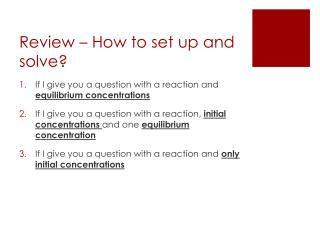DownloadDownload PresentationReview – How to set up and solve?

# Review – How to set up and solve?

Télécharger la présentation## Review – How to set up and solve?

- - - - - - - - - - - - - - - - - - - - - - - - - - - E N D - - - - - - - - - - - - - - - - - - - - - - - - - - -
##### Presentation Transcript

1. Review – How to set up and solve? If I give you a question with a reaction and equilibrium concentrations If I give you a question with a reaction, initial concentrations and one equilibrium concentration If I give you a question with a reaction and only initial concentrations

2. Homework Answers (CHEM 12 TABLE QUESTIONS USING THE QUADRATIC FORMULA) 1.) x = 0.221 Therefore the equilibrium concentrations are: [PCl3] = 0.221M [Cl2] = 0.221M [PCl5] = 0.779M 2.) x = 0.261 Therefore the equilibrium concentrations are: [COCl2] = 0.261M [CO]= 0.239M [Cl2] = 0.739M

3. Question 1: Calculate all three equilibrium concentrations when Keq is equal to 16.0 and the initial concentration of PCl5 in 1.00M. PCl3 + Cl2 PCl5

4. Question 2: Calculate all three equilibrium concentration when Keq is equal to 0.680 and the initial concentrations of CO and Cl2 are 0.500M and 1.00M respectively. COCl2 CO +Cl2

5. LeChatelier’s Principle • A system at dynamic equilibrium can be disrupted by an applied stressed on the system • “If a system at equilibrium undergoes a change, the system will shift to relieve the stress” - Henri Le Chatelier

6. LeChatelier’s Principle The three types of stresses include changes in: 1. Concentration 2. Temperature 3. Pressure

7. Concentration A + 2B  C + D What would happen if the concentration of A is increased? • Equilibrium would shift to the right (towards the products) What would happen if the concentration of B is decreased? - Equilibrium would shift to the left (towards the reactants

8. Temperature A + 2B  C + D Exothermic reaction (ΔH = negative) If this system is at equilibrium at 300°C, what would happen to the equilibrium if the temperature was increased to 500°C? - In order to counterbalance this change – the system will need to absorb heat to lower the temperature. Therefore the system will shift to the left (reactants) If this system is at equilibrium at 300°C, what would happen to the equilibrium if the temperature was decreased to 200°C - In order to counterbalance this change – the system will need to produce heat to raise the temperature. Therefore the system will shift to the right (products)

9. Pressure • ONLY APPLIES TO REACTIONS INVOLVING GASES A(g) + 2B(g)C(g) + D(g) What would happen if the pressure was increased? • Equilibrium would shift towards the side with fewer moles, therefore the reactants What would happen id the pressure was decreased? - Equilibrium would shift towards the side with more moles, therefore the products